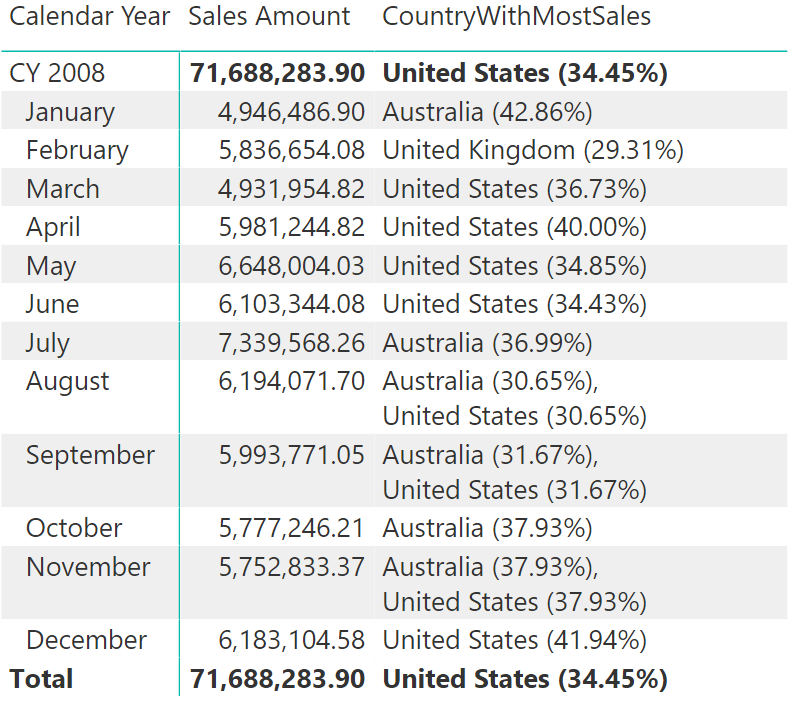In this example, using CONCATENATEX helps identify – out of a list of countries where a company does business – the top performing country with its relative sales volume. The goal is to obtain the following report:There are a few challenges to consider in writing this measure:

• The measure must return the top performing country based on sales.
• The measure must return a string, not an aggregated number.
• The result needs to contain both country name and sales percentage.
• If several countries are in a tie, the measure must return all countries involved. It cannot just return one country – the first country in alphabetical order.

This is the complete measure code:

```CountryWithMostSales :=
VAR TotalSales = [RoundedSales]
VAR CountrySales = ADDCOLUMNS ( VALUES ( Customer[CountryRegion] ), "Sales", [RoundedSales] )
VAR CountryPerc = ADDCOLUMNS ( CountrySales, "Perc", DIVIDE ( [Sales], TotalSales ) )
CountryPerc,
"Result", Customer[CountryRegion] & " (" & FORMAT ( [Perc], "0.00%" ) & ")"
)
RETURN
IF (
TotalSales > 0,
CONCATENATEX (
SELECTCOLUMNS (
TOPN ( 1, Results, [Sales] ),
"Result", [Result]
),
[Result],
CONCATENATE ( ",", UNICHAR ( 10 ) )
)
)
```

The calculation follows the following steps:

1. TotalSales contains the amount of sales for the current cell. It is worth noting that this example uses a rounded amount for sales for the sole purpose of forcing ties – using the exact sales value would not allow for ties and the demo would be less effective.
2. CountrySales stores the country names along with sales volume in each country.
3. CountryPerc adds country sales percentages against TotalSales.
4. Results is the last table variable. It adds a new column combining country name and percentage as a new string, following the format “United States (25.00%)”

At this point, extracting the first row from Results which would contain the string to produce in the report, is sufficient. TOPN is the function extracting that row, but there is a major drawback here: in case of a tie, TOPN returns all values involved.

In case TOPN returns multiple rows, it is necessary to show them one after the other so to make it clear that the result is not unique. CONCATENATEX is an iterator that concatenates strings and produces a single string out of a table. The third parameter of CONCATENATEX is the separator between the elements concatenated. In this very specific case, it is a comma followed by UNICHAR(10), which is nothing but a complex way of writing “please add a new line” in DAX.

CONCATENATEX is very useful in scenarios like this one, where it is not possible to assume that the result is a table of a single row. DAX treats a table with one row and one column as a scalar value. Indeed, VALUES is oftentimes used to retrieve a value out of a singleton table. Nevertheless, CONCATENATEX provides a more robust solution because it also works whenever the table contains multiple rows.

CONCATENATEXEvaluates expression for each row on the table, then return the concatenation of those values in a single string result, seperated by the specified delimiter.

`CONCATENATEX ( <Table>, <Expression> [, <Delimiter>] [, <OrderBy_Expression> [, [<Order>] [, <OrderBy_Expression> [, [<Order>] [, … ] ] ] ] ] )`

TOPNReturns a given number of top rows according to a specified expression.

`TOPN ( <N_Value>, <Table> [, <OrderBy_Expression> [, [<Order>] [, <OrderBy_Expression> [, [<Order>] [, … ] ] ] ] ] )`

UNICHARReturns the Unicode character that is referenced by the given numeric value.

`UNICHAR ( <Number> )`

VALUESWhen a column name is given, returns a single-column table of unique values. When a table name is given, returns a table with the same columns and all the rows of the table (including duplicates) with the additional blank row caused by an invalid relationship if present.

`VALUES ( <TableNameOrColumnName> )`